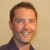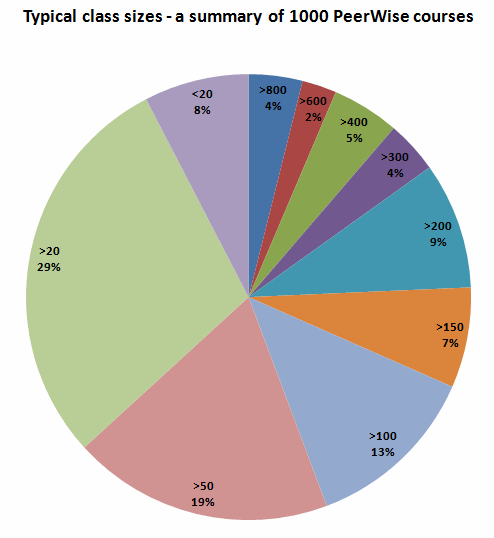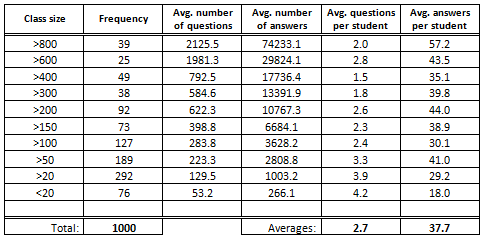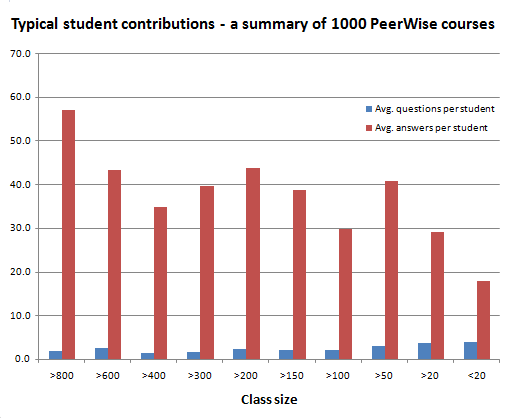# Community posts

## What does a typical PeerWise course look like?by Paul Denny
The University of Auckland

If you have ever wondered whether your class is too small (or too big) to use a tool like PeerWise, you may be interested in the following data. To get a sense for both the typical size of a class on PeerWise, and the typical number of contributions made by students in each class, data from the last 1000 courses was examined.

While there are many examples of very large classes (>300 students), and even a few extremely large ones (>800 students), the majority of classes have fewer than 50 students. The breakdown is given in the chart below.In terms of student participation, it is quite common for instructors to reward a small fraction of course credit to students who contribute at least a certain number of questions and answers (for example, in the most recent class I taught, students were required to author 2 and answer 20 questions).

The table below gives the average overall participation (in terms of questions and answers) for classes of different sizes. It also shows the average contributions per student in each of those classes.The rightmost columns of this table are perhaps the most interesting - the chart below plots the figures from these rightmost columns - that is, the average number of questions authored and the average number of questions answered, by students in classes of various sizes.If you ignore the very small (<20) and very large (>800) classes, the average number of answers submitted by students in all courses falls in quite a narrow range - from just below 30 to just above 40 answers per student. Unsurprisingly, the average number of answers drops in very small courses - as students in these courses are unlikely to have a very large number of questions available to them to answer. Conversely, very large courses sometimes end up with very large banks of questions (often many thousands of questions), enabling the very enthusastic students to answer (almost) as many questions as they would like.

So, where does your class fit in, and could the contributions of your students be described as "typical"?